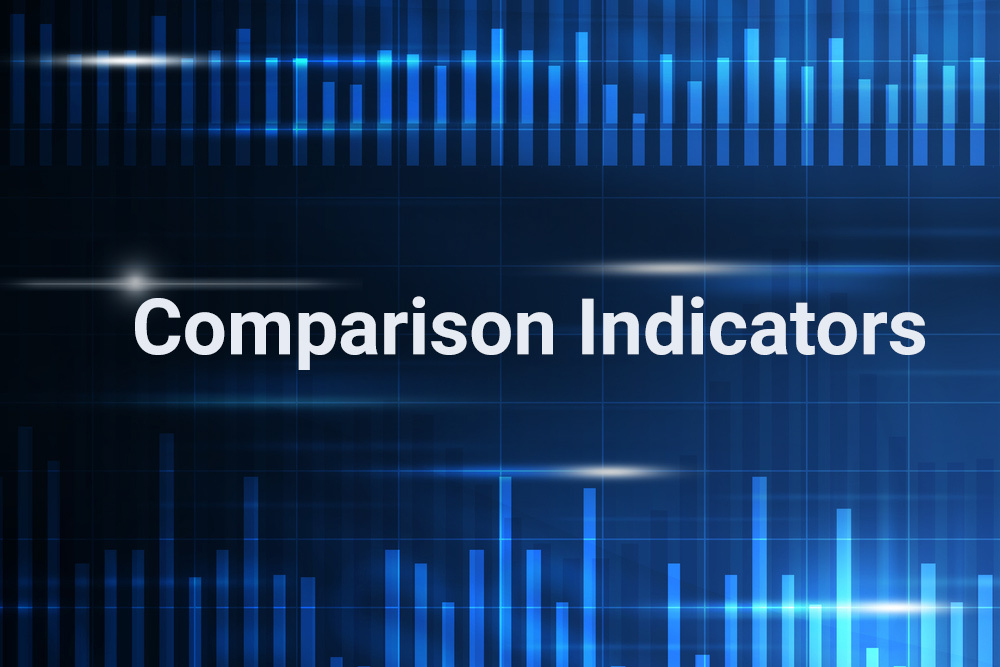# The Complete Guide to Comparison Indicators

The comparison indicator is a technical tool that analyzes relationships between two or more securities, indices, or markets. It compares prices, volume, and/or volatility, determining which instrument is relatively stronger or weaker over the chosen time frame. This type of analysis elicits a series of convergence and divergence signals, with the leading instrument generating a bullish divergence when it gets even stronger compared to the lagging instrument, and a bearish convergence when it gets even weaker compared to the lagging instrument.

This type of analysis may also measure correlation, which is the tendency of one security or indicator to mimic the behavior of another security or indicator. Correlation analysis first measures the relationship between two securities, or a security and a technical indicator. The resulting values are then strung together in a new indicator that visualizes how changes in one variable over time influence changes in the other variable.

This plot allows the technician to predict how price behavior in a correlated market will influence investment or trading returns. When computing correlation, one security or indicator is considered to be the dependent variable while the other security or indicator is considered to be the independent variable.  The calculation determines if a change in the independent variable is expected to result in a similar change in the dependent variable.

## Plot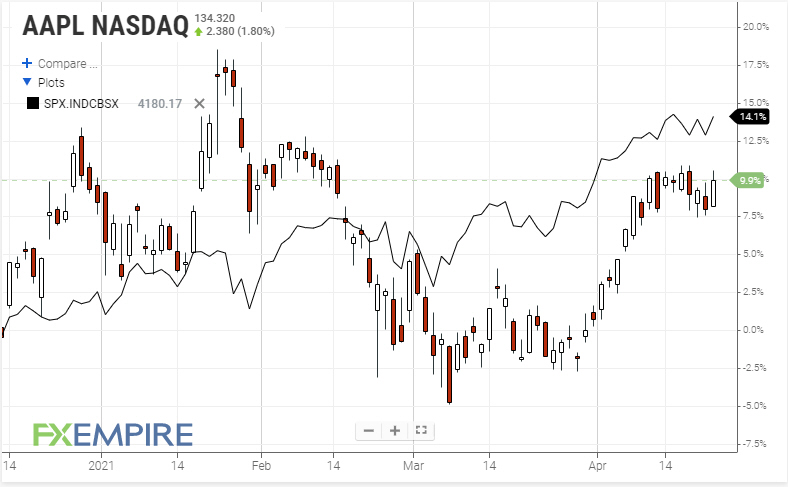The Plot function simply adds a second security to an Advanced Chart price panel and examines relative strength and weakness between instruments. This built-in charting function normalizes divergent security behavior by replacing Y-axis prices with percentage change. The first security becomes the independent variable when performing this analysis while the second security acts as the dependent variable and can be substituted with other securities, as needed.

Comparison and correlation analysis can be accomplished in several ways with the Plot function. The easiest method just scans price action over days, weeks, or months, looking for relative highs and lows to occur at the same time. Crossovers between securities are especially useful in this analysis because it identifies turning points and divergences, in which one security shifts from a leading into a lagging relationship with the other security. The Plot function is used in conjunction with the Correlation Coefficient and Performance Index indicators.

## Correlation Coefficient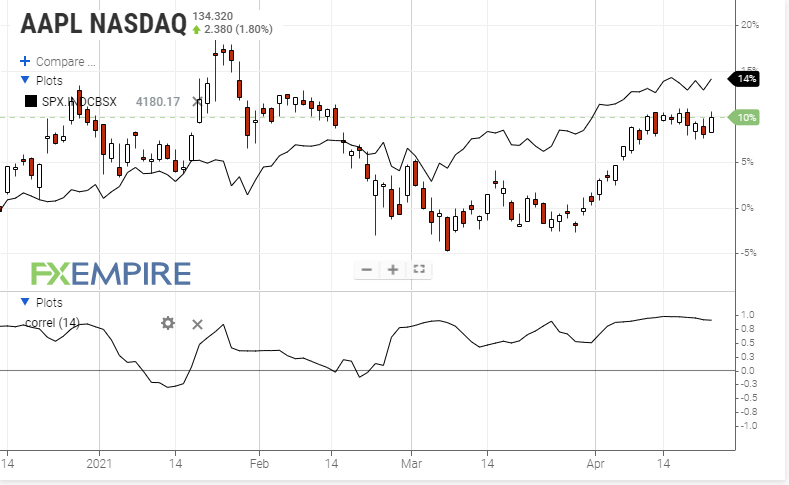Correlation Coefficient indicator evaluates the relationship between a security price and a related technical indicator, or two securities. As noted above, correlation is measured by choosing an independent variable and comparing performance against a dependent variable, which is usually a related security. Indicator output ranges from plus 1 to minus 1 (+1 to -1), with perfect positive correlation at the upper limit and perfect negative correlation at the lower limit.

A perfect positive reading specifies that any change in the independent variable will generate an identical change in the dependent variable. Conversely, a perfect negative reading specifies that any change in the independent variable will generate an identical but opposite change in the dependent variable.  Not surprisingly, a correlation coefficient of 0 specifies there is no relationship or correlation between the two variables.

The direction of the dependent variable’s plot over time depends on whether the coefficient is positive or negative. When the coefficient is positive, the dependent variable will move in the same direction as the independent variable. Conversely, when the coefficient is negative, the dependent variable will move in the opposite direction of the independent variable. Choosing an appropriate holding period is critical when applying this analysis to trade management because correlation tends to oscillate over time, generating frequent whipsaws.

## Performance Index (PI)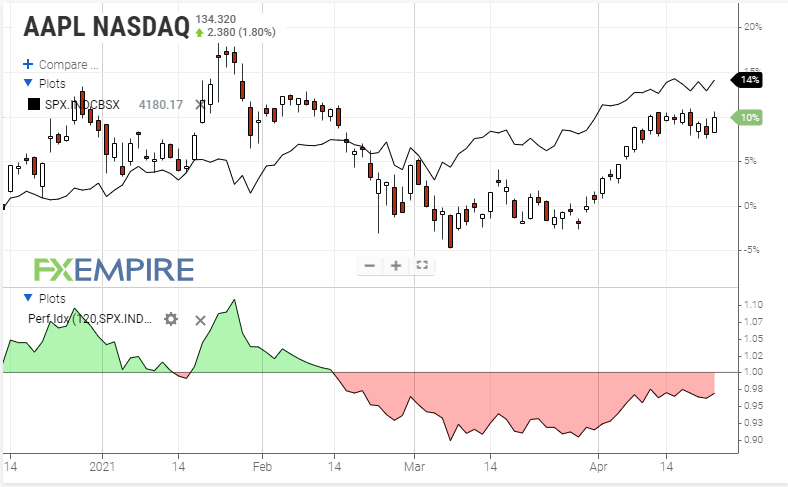Performance Index is used in conjunction with the Plot function. The indicator compares a security’s price to a benchmark index or other security, generating a line that turns green above a reading of 1.0 and red below a reading of 1.0. A reading of exactly 1.0 indicates perfect correlation with the underlying security or index. A security that outperforms a benchmark index should generate higher returns in a trend-following strategy than a security that underperforms a benchmark index.

A rising PI indicates the security is making a stronger move to the upside than the underlying index, or the security is rising while the underlying index is falling. A declining PI indicates the security is declining at a faster rate than the underlying index, or the security is falling while the underlying index is rising.  Flat PI readings indicate that both markets are gaining or losing value at an equal pace.

## Price Relative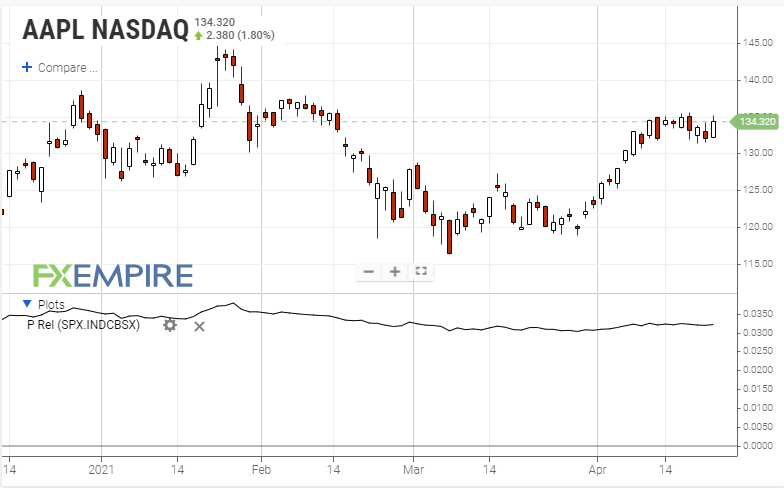Price Relative indicator compares the performance of a security against an underlying index, sector, or another security through a ratio chart.  The indicator is also called the Relative Strength indicator, not to be confused with Wilder’s Relative Strength Index (RSI). This analysis generates convergence and divergence signals that may predict relative returns on active positions over time.

It is a simple plot, calculated by taking the closing price of a dependent variable (base security) and dividing it by the closing price of an independent variable (comparative security). A rising ratio indicates the base security is rising at a faster pace than the comparative security, or falling at a slower rate than the comparative security. Conversely, a falling ratio indicates the base security is falling at a faster pace than the comparative security, or rising at a slower pace than the comparative security.